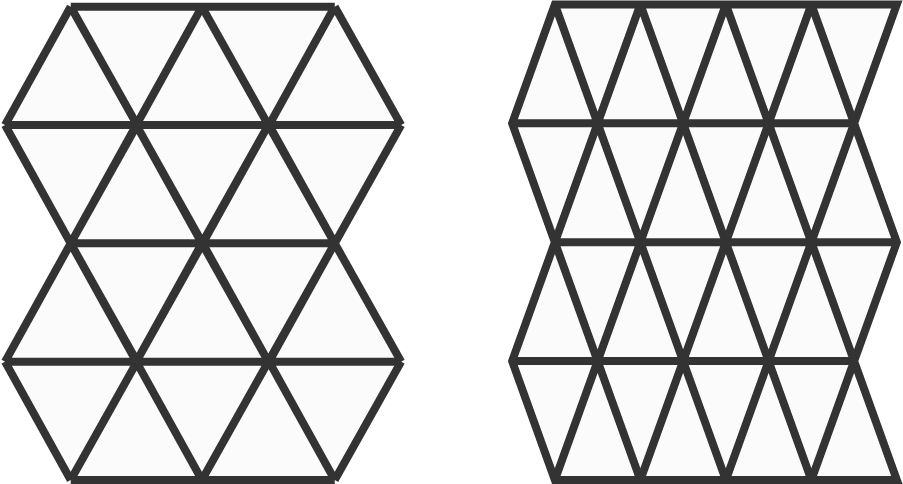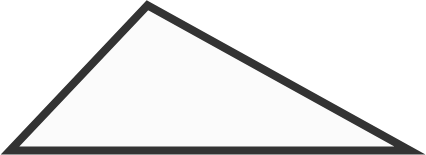# Tessellating with Triangles

Geometry Level 1

An infinite plane can be tiled with identical equilateral or isosceles triangles with no overlaps and no gaps.Is it possible to tile an infinite plane with identical scalene triangles (identical triangles with distinct side lengths)?Note: Once you find a way to put the tiles together to cover a small area—as in the two examples shown—you can assume that the same method can cover an infinite plane.

×